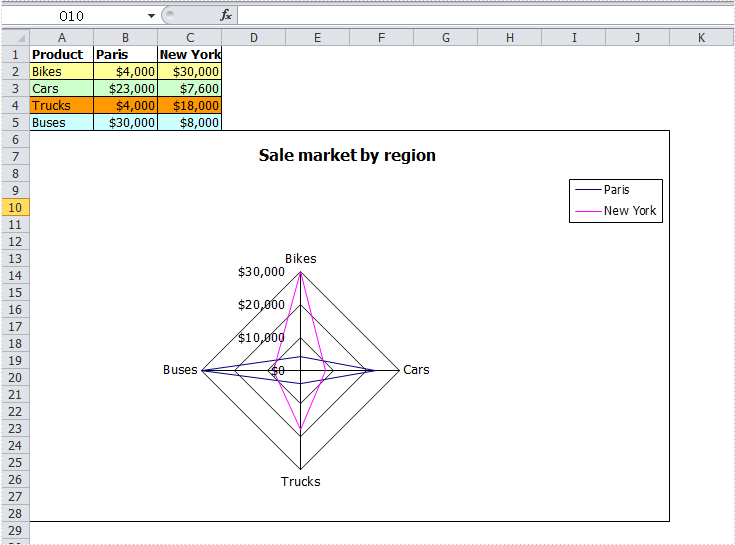# Create Excel Radar Chart in C#, VB.NET

Radar chart is one kind of excel charts. It is also known as spider chart, star chart, cobweb chart, web chart, star plot, irregular polygon, polar chart, or kiviat diagram which is a graphical method of displaying multivariate data in the form of a two-dimensional chart of three or more quantitative variables represented on axes starting from the same point.

Radar chart is very useful to display multivariate observations with an arbitrary number of variables. Each star represents a single observation. Typically, radar charts are generated in a multi-plot format with many stars on each page and each star represents one observation.

### How to Use C# Create Excel Radar Chart

Spire.XLS allows user to create kinds of charts in Excel including Radar Chart. It is easy to create Excel Radar chart with C# via Spire.XLS as in MS Excel. The whole process is almost the same such as write chart data, set region/position of chart, Write chart title, chart data information, etc. The difference is we need fill these data in C# application with codes around. The following is a sample for C# code used to create Excel Radar charts. Download Spire.XLS (or Spire.Office) with .NET framework 2.0 (or above) together and use the code to create Excel Radar Chart right now.

[C#]
```		private void btnRun_Click(object sender, System.EventArgs e)
{
Workbook workbook = new Workbook();

//Initailize worksheet
workbook.CreateEmptySheets(1);
Worksheet sheet = workbook.Worksheets;
sheet.Name = "Chart data";
sheet.GridLinesVisible = false;

//Writes chart data
CreateChartData(sheet);
//Add a new  chart worsheet to workbook

//Set position of chart
chart.LeftColumn = 1;
chart.TopRow = 6;
chart.RightColumn = 11;
chart.BottomRow = 29;

//Set region of chart data
chart.DataRange = sheet.Range["A1:C5"];
chart.SeriesDataFromRange = false;

if (checkBox1.Checked)
{
}
else
{
}

//Chart title
chart.ChartTitle = "Sale market by region";
chart.ChartTitleArea.IsBold = true;
chart.ChartTitleArea.Size = 12;

chart.PlotArea.Fill.Visible = false;

chart.Legend.Position = LegendPositionType.Corner;
workbook.SaveToFile("Sample.xls",ExcelVersion.Version97to2003);
ExcelDocViewer(workbook.FileName);
}

private void CreateChartData(Worksheet sheet)
{
//Product
sheet.Range["A1"].Value = "Product";
sheet.Range["A2"].Value = "Bikes";
sheet.Range["A3"].Value = "Cars";
sheet.Range["A4"].Value = "Trucks";
sheet.Range["A5"].Value = "Buses";

//Paris
sheet.Range["B1"].Value = "Paris";
sheet.Range["B2"].NumberValue = 4000;
sheet.Range["B3"].NumberValue = 23000;
sheet.Range["B4"].NumberValue = 4000;
sheet.Range["B5"].NumberValue = 30000;

//New York
sheet.Range["C1"].Value = "New York";
sheet.Range["C2"].NumberValue = 30000;
sheet.Range["C3"].NumberValue = 7600;
sheet.Range["C4"].NumberValue = 18000;
sheet.Range["C5"].NumberValue = 8000;

//Style
sheet.Range["A1:C1"].Style.Font.IsBold = true;
sheet.Range["A2:C2"].Style.KnownColor = ExcelColors.LightYellow;
sheet.Range["A3:C3"].Style.KnownColor = ExcelColors.LightGreen1;
sheet.Range["A4:C4"].Style.KnownColor = ExcelColors.LightOrange;
sheet.Range["A5:C5"].Style.KnownColor = ExcelColors.LightTurquoise;

//Border
sheet.Range["A1:C5"].Style.Borders[BordersLineType.EdgeTop].Color = Color.FromArgb(0, 0, 128);
sheet.Range["A1:C5"].Style.Borders[BordersLineType.EdgeTop].LineStyle = LineStyleType.Thin;
sheet.Range["A1:C5"].Style.Borders[BordersLineType.EdgeBottom].Color = Color.FromArgb(0, 0, 128);
sheet.Range["A1:C5"].Style.Borders[BordersLineType.EdgeBottom].LineStyle = LineStyleType.Thin;
sheet.Range["A1:C5"].Style.Borders[BordersLineType.EdgeLeft].Color = Color.FromArgb(0, 0, 128);
sheet.Range["A1:C5"].Style.Borders[BordersLineType.EdgeLeft].LineStyle = LineStyleType.Thin;
sheet.Range["A1:C5"].Style.Borders[BordersLineType.EdgeRight].Color = Color.FromArgb(0, 0, 128);
sheet.Range["A1:C5"].Style.Borders[BordersLineType.EdgeRight].LineStyle = LineStyleType.Thin;

sheet.Range["B2:C5"].Style.NumberFormat = "\"\$\"#,##0";
}
```

After running your application with the code above, you will find an Excel Radar Chart created.